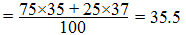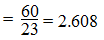## Latest Articles

Atomic Weight is the average relative weight per atom of an element according to their abundance considering an atom of C12 isotopes as 12 amu.
For Example:
Naturally occurring Chlorine contains 75% Cl35 and 25% Cl37, so atomic of ChlorineIn other words, atomic weight is the number which show how many times an atom of an element is heavier that 1/12th part of an atom of  12Cl isotopes.

Fractional Atomic Weight:
Atomic Weight is the average  weight per atom of an element. So it can be in fraction due to the presence of isotopes.
For Example:
Naturally occurring Chlorine contains 75% Cl35 and 25% Cl37, so atomic of ChlorineGram Atomic Weight:
When atomic weight of an element is expressed in gram. Then it is called gram atomic weight. One gram atomic weight of each element contains 6.023×1023 atoms.

For eg.
Atomic Weight of Sodium = 23
23 gram sodium = 1 gram atom of sodium
=  6.023×1023 atoms of sodium

Q. Calculate the number of gram atoms and atoms of sodium present in 60 gram of sodium.
Solution:
Given,
Weight of Sodium = 60 gram
Atomic Weight of Sodium = 23
No. of gram atoms of sodium = ?
No. of atoms of sodium = ?1 gram atom = 6.023×1023 atoms
2.608 gram atoms = 6.023×1023×2.608 atoms
= 1.57×1024 atoms

Q.What is the weight of one atom of sodium?
Solution:
Atomic Weight of sodium = 23
23 gram of sodium = 1 gram atom of sodium
= 6.023×1023 atoms of sodium
6.023×1023 atoms of sodium = 23 gram

= 3.81×10-23 gram.

Q.What is the weight of 1 amu carbon?
Atomic weight of carbon = 12
12 gram of carbon = 1 gram atom of carbon
=  6.023×1023atoms of carbon
= 6.023×1023×12 amu

6.023×1023×12 amu = 12 gram